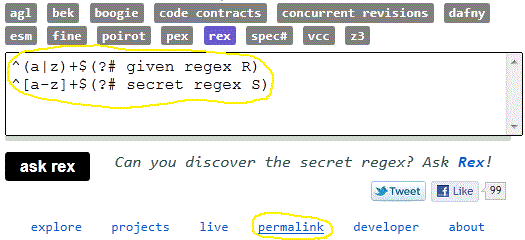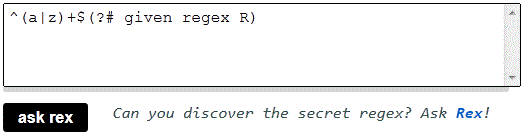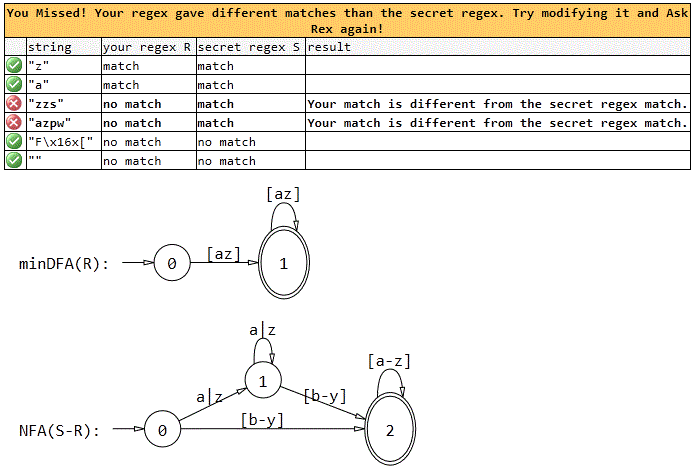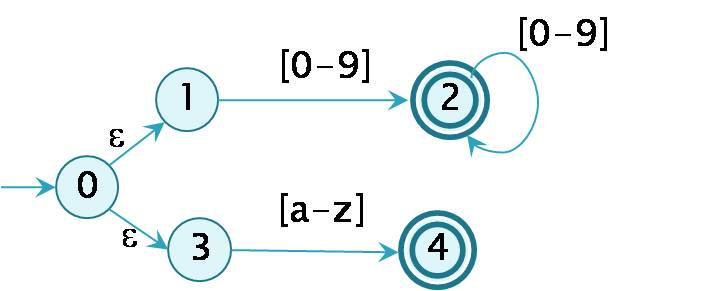# Rex – Regular Expression Exploration

Established: March 27, 2010

Rex is a tool that explores .NET regexes and generates members efficiently.

# Try out Rex in duel mode at http://rise4fun.com/rex!

The duel mode is a game where you have to guess a secret (hidden) regex. On each attempt, Rex generates strings that match or don’t match the same way as the secret regex. The game uses the ASCII range of characters, i.e. characters from code 0 to 127 and displays various automata associated to the regexes.

## How does the duel work?

There is a secret regex S and a given regex R. When you ask rex, a table is displayed showing (up to two) members of

• L(R) intersect L(S),
• L(R) minus L(S),
• L(S) minus L(R), and
• complement(L(R) union L(S)),

where L(r) denotes the set of all strings matching a regex r. In addition, the following automata are shown for R:

• (e)NFA: Nondeterministic Finite Automaton (with epsilon moves),
• (min)DFA: (minimal) Deterministic Finite Automaton

All automata are displayed in symbolic or extended form, meaning that the labels on the transitions denote (nonempty) sets of characters rather than individual characters.

The duel also displays an NFA accepting L(S) minus L(R), denoted by NFA(S-R).

Duels uses features of the underlying automata library (used by Rex) such as:

• construction of symbolic finite automata (SFAs) from .NET regexes
• difference construction of SFAs
• equivalence checking of SFAs
• intersection of SFAs
• complemetation of SFAs
• minimization of SFAs
• visualization of SFAs as directed graphs using MSAGL
1. In http://www.rise4fun.com/rex write two regexes on separate lines, say R and S
2. Click permalink to create a duel where R is visible and S is hidden.This creates a permanent web link containing the duel.

You have created your own duel!

### ExampleThis particular example is the link http://www.rise4fun.com/Rex/abG.When you visit the link, only R is shown, S remains secret:When you now ask rex, the following results are shown:Most regex constructs are explained in .NET regexes.

### Using character classes

Regarding character classes, a less known but useful construct is subtraction operation in character classes:

• [character_class-[character_class]]

When writing regexes, you can insert comments, e.g., to provide a hint in the given regex in the duel, for solving the secret regex

• (?# comment text)

### Examples:

• [a-z-[d-g]](?# the set of all lowercase letters except d, e, f and g)note the two different meanings of -, used in this character class
• [w-[d]](?# the set of all word characters except digits)e.g., [w-[d]] is equivalent (in ASCII range) to [a-zA-Z_] or [w-[0-9]]
• [a-[a]](?# the empty set of characters)
• ^[a-z]+\$(?# regex matching one or more lowercase letters)
• ^d{2,}\$(?# regex matching two or more decimal digits)e.g., ^d{2,}\$ is equivalent to ^[0-9]{2,}\$ or ^dd+\$
• Rex is a simple command line tool that takes a .NET regex pattern or several regex patterns and generates matching string(s) for them. Rex can also be used through an API. Rex is fast, using a novel approach to solve this problem. When several regexes are provided, rex can be used to generate members that match all the regexes. By default, rex assumes UTF16 encoding of characters, but can also be restricted to ASCII ecoding.

The following example generates 10 strings that start and end with a digit and have at least two characters:

`> rex.exe "^d.*d\$" /k:10`

In order to produce members that contain only ascii characters the /e option can be used. For general instructions regarding usage run:

`> rex.exe /?`
• 1) A regex pattern (or several patterns) is translated into a symbolic finite automaton

^[0-9]+|[a-z]\$   ==>2) The automaton is given to a constraint solver that generates members from it.

## People### Peli de Halleux

Principal RSDE### Margus Veanes

Principal Researcher